NSP-111-200927-052815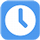Please Do not press "Submit" at the end of the exam until you are sure of your responses, as your test will be graded immediately.
You can hover your mouse pointer over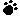to see the question ID. Good luck!

1.Round off 4 213 to the nearest hundred.

2.There were some cattle and sheep in a zoo. There were twice as many sheep as cattle. The zoo keeper counted 84 legs altogether. How many cattle were there?

3.Meena bought 1kg of onions which cost ＄1.80 and 1kg of cucumbers which cost twice as much as the onions. She gave ＄10 to the vegetable seller. How much change did she receive?

4.Mandy and Samuel have ＄460. Mandy has 4 times as much as Samuel. How much does Mandy have?

5.A bowling alley opens at 11.30 am and closes at 5 pm every day. How long is it opened each day?

6.3 420 ÷ ? = 342 The missing number in the box is_____________.

7.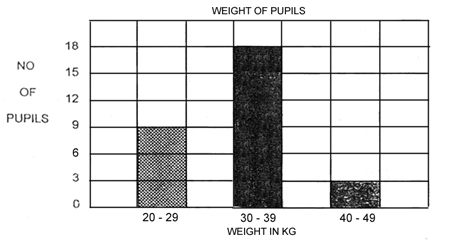The number of pupils who weigh between 30-39 kg is twice the number of pupils who weigh between 20-29 kg. How many pupils weigh between 30-39 kg?

8.I am 9 years and 10 months old. My sister is 1 year 3 months older. How old is my sister?

9.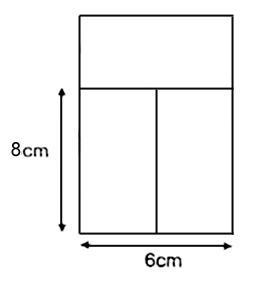The figure below is made up of 3 rectangles. Find the area of the whole figure. (The figure is not drawn to scale)

10.Ali took 6 minutes to draw a horse. He took twice as long to draw a dragon. He then, took four times as long to draw a castle with a dragon in it. How long did he take to draw the castle with a dragon in it?

11.? - 453 = 545 The missing number in the box is __________________. Round off your answer to the nearest 10.

12.Find the product of 96 and 78.

13.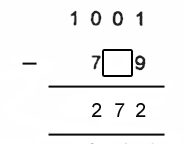The missing number in the box is ______________.

14.? ÷ 3 = 64 R2
The missing number in the box is ________________

15.When 564 is divided by 7, the remainder is ________________.

16.Andrew has ＄240.
Andrew has 6 times as much as Jane.
How much more money does Andrew have than Jane?

17.Arrange the digits 4, 7 and 2 to form the GREATEST EVEN number. The number MUST be a multiple of 4.

18.There were 2145 children at a concert. There were 348 fewer adults than children at that concert. How many adults were there at the concert?

19.If 72 is the ninth multiple of a certain number, what is that number?

20.Carl has 13 toy cars more than Jack. Jack has 5 toy cars less than Simon. How many more toy cars has Carl than Simon?

21.The cost of tickets to a show was ＄11 for 1 adult and ＄7 for 1 child. Mrs Tang bought tickets for 4 adults and 5 children. If she gave the cashier ＄100, how much change would she receive?

22.There are 360 pupils in the hall. Mr Lee wants to arrange them in groups. Mr Lee puts 9 pupils in each group. How many groups are there?

23.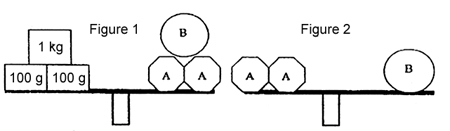What is the mass of A?

24.Multiply 34 by 21. The answer is _______________ when rounded off to the nearest ten.

25.Find the difference between 4 359 and 897.

26.How many hundreds are there in 23 000?

27.A factory can manufacture 32 pairs of boots a day. In 14 days, how many pairs of boots can it manufacture?

28.4 560 is 500 more than _______________.

29.A given number is a multiple of 5. It is between 20 and 30. It is also a factor of 50. What is the number?

30.Which of the following has the smallest value?